# Preface

The Gibbs phenomenon was first noticed and analyzed by the English mathematician Henry Wilbraham (1825--1883) in 1848, and rediscovered by an American scientist J. Willard Gibbs (1839--1903) 50 years later. The term "Gibbs phenomenon" was introduced by the American mathematician Maxime Bocher in 1906. The history of this discovery can be found in an article by Hewitt&Hewitt. This phenomenon assures that the Fourier series of a piecewise continuously differentiable periodic function oscillates near the jump of discontinuity by overshooting and undershooting it by about 9%. This lack of improvement in the approximations near the discontinuity manifested in the continual presence of the overshoot or undershoot is called the Gibbs phenomenon. Henry Wilbraham received a BA in 1846 and an MA in 1849 from Cambridge.

Introduction to Linear Algebra with Mathematica

# Gibbs Phenomenon

Let $$F_N (x)$$ be the finite Fourier sum for the periodic function f(x) with N+1 terms:

$F_N (x) = \frac{a_0}{2} + \sum_{k=1}^N \left( a_k \cos \frac{k\pi x}{\ell} + b_k \sin \frac{k\pi x}{\ell} \right) ,$
where the coefficients 𝑎n and bn were defined previously. The Gibbs phenomenon is the peculiar manner in which the Fourier series of a piecewise continuously differentiable periodic function behaves at a jump of discontinuity. The partial Fourier sums ripple near every point of discontinuity in an amount proportional to the finite jump. So finite Fourier sums (as well as other eigenfunction series) provide an overshoot/undershoot (or "ringing") occurring at discontinuities of finite length. Mathematically, it reflects the difference between uniform convergence and pointwise convergence. The Nth partial sum of the Fourier series has large oscillations near the jump, which increases/decreases the maximum/minimum of the partial sum of the function itself. The overshoot does not die out as N increases, but instead approaches a finite limit. If a function f(x) has a discontinuity at the point $$x_0$$ of amount $$c = f(x_0 +0) - f(x_0 -0) ,$$ then finite Fourier sums experience overshoot and undershoot in a neighborhood of the amount $$c\, w ,$$ where w = 0.0894899… is the Wilbraham constant. These undershoots or overshoots cannot be eliminated by increasing the number of terms in the finite Fourier partial sums; actually these finite sums swap the range
$\frac{2}{\pi}\,\mbox{Si}(\pi ) \times \left\vert f(x_0 +0) - f(x_0 -0) \right\vert = 1.1789797444721675\ldots \times \left\vert f(x_0 +0) - f(x_0 -0) \right\vert$
when $$N\mapsto \infty .$$ Here Si is the sine integral
$\mbox{Si}(x) = \int_0^{x} \frac{\sin t}{t}\,{\text d}t ,$
a special function that can be evaluated by Mathematica with a one line build-in command
N[SinIntegral[Pi]]
1.851937051982466
Division by π/2
N[Pi/2]
1.5707963267948966
yields
N[SinIntegral[Pi]/Pi*2]
1.1789797444721672
So the overshoot/undershoot of a finite Fourier series for a function having a unit jump of discontinuity will exceed the actual value of the function at that point by the Wilbraham constant
$w = \frac{1}{2} \left( \frac{2}{\pi}\,\mbox{Si}(\pi ) -1 \right) = \frac{1}{2} \left( \frac{2}{\pi}\,\int_0^{\pi} \frac{\sin t}{t}\,{\text d}t -1 \right) \approx 0.08948987223608362 ,$
which is evaluated with 20 decimale places by Mathematica as
w = N[(SinIntegral[Pi]/Pi*2 - 1)/2, 20]
0.089489872236083635116

If the function is continuous (actually, its periodic continuation on all real axis), we do not observe the Gibbs phenomenon. The considered examples show that if a function is a piecewise continuous with finite jumps, its Fourier coefficients decay at a rate of 1/k; if a continuous function has corners (not differentiable), then its Fourier coefficients decay at a rate of 1/k2. The more derivatives exist, the faster Fourier coefficients decay. The general case provides the following lemma.

If a periodic function f of period T is absolutely integrable (so $$\int_{T} \left\vert f(x) \right\vert {\text d}x < \infty ,$$ then its Fourier coefficients tend to zero.

# Derivation of Wilbraham constant

Example 1: Consider a piecewise continuous function (which is actually piecewise constant):

$f(x) = \begin{cases} 1 , & \quad \mbox{if x\in (-2,-1), } \\ 0 , & \quad \mbox{if x\in (-1,0), } \\ 2, & \quad \mbox{for }x\in (0,2) . \end{cases}$
We calculate its Fourier coefficients with Mathematica:

x[t_]= Piecewise[{{1,-2<t<-1},{0,-1<t<0},{1,0<t<2}}]
a0=Integrate[1,{x,-2,-1}]/2 + Integrate[1,{x,0,2}];
an = Integrate[Cos[n*Pi*x/2], {x, -2, -1}] /2 + Integrate[Cos[n*Pi*x/2], {x, 0, 2}];
bn = Integrate[Sin[n*Pi*x/2], {x, -2, -1}] /2 + Integrate[Sin[n*Pi*x/2], {x, 0, 2}];
fourier[m_] = 5/4 + Sum[an*Cos[n*Pi*x/2] + bn*Sin[n*Pi*x/2], {n, 1, m}];
Plot[{fourier, fourier}, {x, -2.1, 2.1}, PlotStyle -> {{Thick, Blue}, {Thick, Orange}}, Ticks -> {{-2, -1, 1, 2}, {2.18, 1.09, 0.91, -0.18}}]
$a_0 = \frac{1}{2} \,\int_{-2}^{-1} {\text d}x + \int_0^2 {\text d}x = \frac{5}{2} , \quad a_n = - \frac{1}{n\pi}\,\sin\frac{n\pi}{2} , \quad b_n = \frac{1}{n\pi} \left[ (-1)^n -2 + \cos \frac{n\pi}{2} \right] .$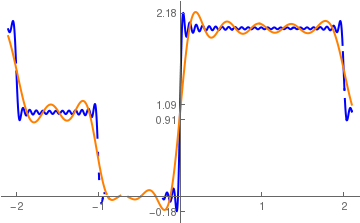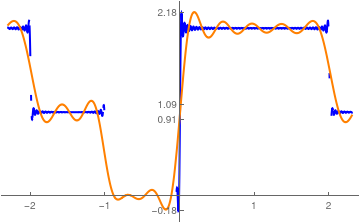Fourier approximation with n=50 vs n=10 terms. Fourier approximation with n=100 vs n=10 terms.

In the graph shown, the orange curve was calculated with n=10 terms while the blue curve was calculated with n=50 terms. As a result, when the number of terms in the Fourier series is increased, the overshoot and undershoot cannot be removed, but the “ripples” will move closer and closer (horizontally) to the point of discontinuity. There are four points of discontinuity on the interval [-2,2]; at three of them (x = -2, -1, 2) the given function experiences jump of discontinuity of 1 and at the point x = 0 it has jump of value 2.

At point x = -2, Fourier series has undershoot value of $$1 - 0.0894899 \approx 0.91051$$ because 0.0894899 ≈ 0.1789797444721675 /2 = 2*Si(π)/π - 1. Correspondingly, at point x = -1, we have overshoot 1 + 0.0894899 ≈ 1.0894899… . At point x = 2, the overshoot will be about 2.0894899 and undershoot will be about 0.91051. However, at point x = 0, the jump of discontinuity is 2; therefore, we expect Gibbs phenomenon at this point to be

• $$1- 1.1789797444721675 \approx - 0.1789797444721675\ldots$$ for undershoot;
• $$2+ 0.1789797444721675 \approx 2.1789797444721675\ldots$$ for overshoot.

Changing the FourierParameters setting allows control over the limits of integration on the coefficients, and therefore, the base frequency of the series. Some work will go into calculating what values for parameters will give the proper limits of integration. There is ordinarily no reason to change the first parameter from its default setting, which is 1.

It has the following Fourier series:

curve5 = FourierTrigSeries[x[t],t,5,FourierParameters->{1,Pi/2}]
Out= 3/4 - Cos[($Pi] t)/2]/\[Pi] + Cos[(3 \[Pi] t)/2]/(3 \[Pi]) - Cos[(5 \[Pi] t)/2]/(5 \[Pi]) + Sin[(\[Pi] t)/2]/\[Pi] + Sin[\[Pi] t]/\[Pi] + Sin[(3 \[Pi] t)/2]/(3 \[Pi]) + Sin[(5 \[Pi] t)/2]/(5 \[Pi]) When plotted, it looks like this: Plot[{x[t],curve5},{t,-2,2}, PlotStyle -> Thick, Ticks-> {{-2,-1,0,1,2},{-0.08,1.08}}]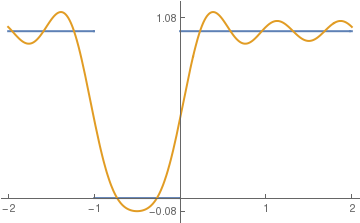Example 2: Consider the continuous function on interval [-π,π]: \[ f(x) = \begin{cases} 0, & \ \mbox{ if } -\pi < x \le 0, \\ x, & \ \mbox{ if } 0 \le x < \Pi . \end{cases}$
It has a Fourier series expansion on [-π,π]:
$f(x) \sim \frac{\pi}{4} - \frac{2}{\pi} \sum_{k\ge 0} \frac{1}{(2k+1)^2} \, \cos \left( (2k+1)x \right) - \sum_{n\ge 1} \frac{(-1)^n}{n} \,\sin nx .$
At point x=π, the function f has a jump of magnitude π≈3.1415926. Therefore, the overshoot will be around
N[Pi*(0.1789797444/2)+Pi]
3.42273
and undershoot similarly
-N[Pi*(0.1789797444/2)]
-0.281141
Now we plot partial sums to check Gibbs phenomenon around points x=-π and x=π:
F[x_]= Pi/4 -2/Pi*Sum[1/(2*k+1)^2 * Cos[(2*k+1)*x], {k,0,10}] - Sum[(-1)^n /n *Sin[n*x], {n,1,21}];
f[x_]= Piecewise[{{0,-Pi < x < 0},{x,0< x < Pi}}];
Plot[{F[x],f[x]},{x,-3.45,3.45}, PlotStyle->Thick, Ticks -> {{-3,-2,-1,1,2,3},{3.42, -0.28}}]
Plot[{F[x], f[x]}, {x, 3.0, 3.45}, PlotStyle -> Thick, Ticks -> {{Pi}, {3.42, -0.28}}]

Example 3: Consider the piecewise continuous function on the interval [-2,2]:

$f(x) = \begin{cases} 1, & \ \mbox {if } -2 < x < -1 , \\ x^2 -1, & \ \mbox {if } -1< x< 2 , \end{cases}$
Its Fourier coefficients were found previously in section:
$f(x) = \frac{1}{4} + \sum_{n\ge 1} \frac{8(-1)^n n\pi + 4n\pi \cos \left( \frac{n\pi}{2} \right) - \left( 8 + n^2 \pi^2 \right) \sin \left( \frac{n\pi}{2} \right)}{n^3 \pi^3} \, \cos \frac{n\pi x}{2} - \sum_{n\ge 1} \frac{2 (-1)^n \left( n^2 \pi^2 -4 \right) + \left( 8 + n^2 \pi^2 \right) \cos \left( \frac{n\pi}{2} \right) + 4n\pi\,\sin \left( \frac{n\pi}{2} \right)}{n^3 \pi^3} \,\sin \frac{n\pi x}{2}$
On the main interval (-2,2], the given function has two jumps of discontinuity:
$\lim_{x\to -1-0} \,f(x) = 1, \quad \lim_{x\to -1+0} \,f(x) = 0; \qquad \lim_{x\to 2-0} \,f(x) = 3, \quad \lim_{x\to 0+0} \,f(x) = 1.$
So, at point x = -1 the function experiences a jump of 1 unit and at the point x = 2 it has a jump of 2 units. Therefore, the undershoot at point x = -1 will be closed to -w ≈ -0.089489872 (Wilbraham number) and overshoot of 1+w ≈ 1.089489872 as the number of terms in partial Fourier sums approaches infinity. At another point of discontinuity x = 2, the given function will have the undershoot of the amount 2w, which will be about 2.82102. Correspondingly, its overshoot will approach 3.1789797. Upon making a computational experiment with plotting partial sums in neighborhoods of these two points, we see that indeed, the predicted overshoots and undershoots are very closed to the predicted ones.
Plot[F10[x], {x, -2.4, 1.4}, PlotStyle -> {Thick, Red}, Ticks -> {{-1}, {-0.089489872, 1.089489872}}]
Plot[F20[x], {x, -1.25, -0.85}, PlotStyle -> {Thick, Orange}, Ticks -> {{-1}, {-0.089489872, 1.089489872}}]
Plot[F100[x], {x, -1.05, -0.95}, PlotStyle -> {Thick, Blue}, Ticks -> {{-1}, {-0.089489872, 1.089489872}}]
Fourier approximation with 10 terms    Fourier approximation with 20 terms    Fourier approximation with 100 terms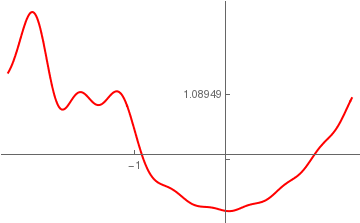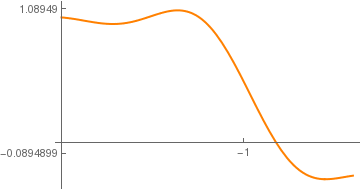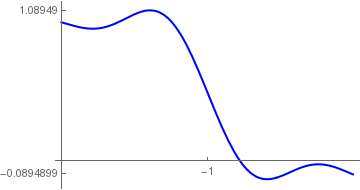Similarly, we plot Fourier series in neighborhoods of another point x = 2.
Plot[F10[x], {x, 1.55, 2.55}, PlotRange -> {{1.55, 2.55}, {0.66, 3.2}}, PlotStyle -> {Thick, Red}, Ticks -> {{2}, {1, 2, 3, 0.8210203, 3.1789797}}]
Plot[F20[x], {x, 1.65, 2.45}, PlotRange -> {{1.65, 2.45}, {0.66, 3.2}}, PlotStyle -> {Thick, Orange}, Ticks -> {{2}, {1, 2, 3, 0.8210203, 3.1789797}}]
Plot[F100[x], {x, 1.95, 2.09}, PlotRange -> {{1.95, 2.09}, {0.66, 3.2}}, PlotStyle -> {Thick, Blue}, Ticks -> {{2}, {1, 2, 3, 0.8210203, 3.1789797}}]
Fourier approximation with 10 terms    Fourier approximation with 20 terms    Fourier approximation with 100 terms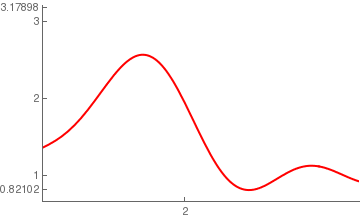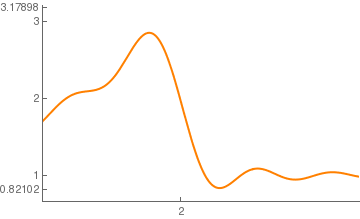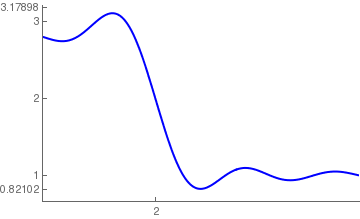■

Example 4: Consider the piecewise signum function on the interval [-π,π]:

$\mbox{sign}(x) = \begin{cases} -1, & \ \mbox {if } -\pi < x < 0 , \\ \phantom{-}1, & \ \mbox {if } 0< x< \pi . \end{cases}$
Since the signum function is odd, we expand it into sine Fourier series
$\mbox{sign}(x) = \sum_{n\ge 1} b_n \sin \left( n\,x \right) ,$
where
$b_n = \frac{2}{\pi} \int_0^{\pi} \sin \left( n\,x \right) {\text d}x = \frac{2}{n\pi} \left( 1 - (-1)^n \right) = \frac{4}{\pi} \times \begin{cases} \frac{1}{2k+1} , & \ \mbox{ if } n = 2k+1 , \\ 0, & \ \mbox{ otherwise.} \end{cases}$
because
Integrate[Sin[n*x], {x, 0, Pi}]*2/Pi
(2 (1 - Cos[n $Pi]]))/(n \[Pi]) First, we plot its Fourier series using standard Mathematica command. ff0[x_] = unitstep[Mod[x, Pi, -Pi]]; f[x_] = Piecewise[{{-1, -Pi < x < 0}, {1, 0 < x < Pi}}]; ft6ff0 = FourierSinSeries[ff0[x], x, 6]; ftff0[x_, nmax_] := Sum[Sin[(2 n + 1) .9 x]/(2 n + 1), {n, 0, nmax}] 1.275 stepplot[n_] := Plot[{f[x], ftff0[x, n]}, {x, -1, 1}, Ticks -> {{1.18, 1, -1.18}}, PlotStyle -> {{Thick, Blue}, {Thick, Red}}, Exclusions -> None] stepplot stepplot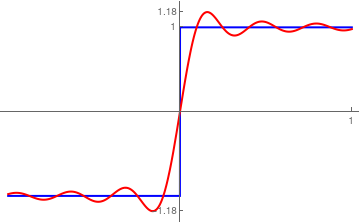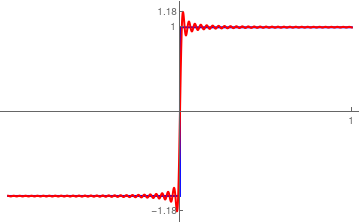Fourier approximation with n=10 terms. Fourier approximation with n=100 terms. Then we verify this series directly \[ \mbox{sign}(x) = \frac{4}{\pi} \,\sum_{k\ge 0} \frac{1}{2k+1}\, \sin \left( (2k+1)\,x \right) .$
The corresponding plot confirms:
g[x_] = 4/Pi*Sum[1/(2*k + 1)*Sin[(2*k + 1)*x], {k, 0, 30}];
Plot[g[x], {x, -2*Pi, 2*Pi}]
Since the signum function has a finite jump of 2 at the origin, the overshoot is about 1.18 (which is 2 times the Wilbraham constant) and undershoot is −1.18.    ■

1. Hewitt, E. and Hewitt, R.E., The Gibbs--Wilbraham phenomenon: An episode in Fourier analysis, Archive for History of Exact Science, 1979, Vol. 21, No. 2, pp. 129--160.
2. B. Kuttner, Note on the Gibbs Phenomenon, Journal of the London Mathematical Society, Volume s1-20, Issue 3, 1 June 1945, Pages 136–139, https://doi.org/10.1112/jlms/s1-20.3.136

A correction has been published: Journal of the London Mathematical Society, Volume s1-20, Issue 4, 1 October 1945, Pages 238, https://doi.org/10.1112/jlms/s1-20.4.238-s